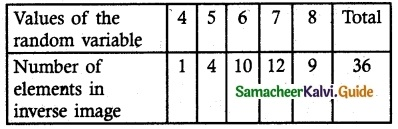Tamilnadu State Board New Syllabus Samacheer Kalvi 12th Maths Guide Pdf Chapter 11 Probability Distributions Ex 11.1 Textbook Questions and Answers, Notes.

## Tamilnadu Samacheer Kalvi 12th Maths Solutions Chapter 11 Probability Distributions Ex 11.1

Question 1.
Suppose X is the number of tails that occurred when three fair coins are tossed once simultaneously. Find the values of the random variable X and number of points in it. inverse – images.
Solution:
Let X is the random variable that denotes the number of tails when three coins are tossed simultaneously.
Sample space S = {HHH, HHT, HTH, HTT, THH, THT, TTH, TTT}
∴ ‘X’ takes the values 0,1, 2, 3
i.e., X (HHH) = 0 ; X (HHT, HTH, THH) = 1 ; X (HTT, THT, TTH) = 2 ; X (TTT) = 3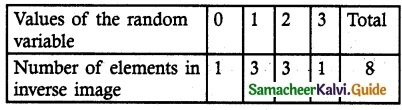Question 2.
In a pack of 52 playing cards, two cards are drawn at random simultaneously. If the number of black cards drawn is a random variable, find the values of the random variable and number of points in its inverse images.
Solution:
Let X be the random variable of number of black cards occur.
∴ X = {0, 1, 2}
The sample space consists of 52C2 = 1326
X = 0, X (both are white cards) = 26C2 = 325
X = 1, X (one black and one white cards)
= 26C1 × 26C1
= 676
X = 2, X (both are black cards) = 26C2 = 325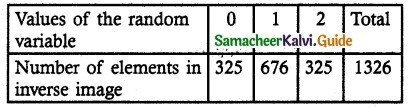Question 3.
An urn contains 5 mangoes and 4 apples. Three fruits are taken at random. If the number of apples taken is a random variable, then find the values of the random variable and number of points in its inverse images.
Solution:
Number of mangoes = 5
Number of Apples = 4
Total number of fruits = 9
Let ‘X’ be the random variable that denotes the number of apples taken, then it takes the values 0, 1, 2, 3
X (MMM) = 0
X (AMM (or) MAM (or) MMA) = 1
X (AAM (or) AMA (or) MAA) = 2
X (AAA) = 3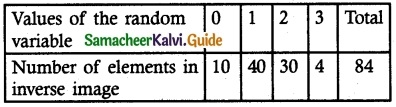Question 4.
Two balls are chosen randomly from an urn containing 6 red and 8 black balls. Suppose that we win Rs 15 for each red ball selected and we lose Rs 10 for each black ball selected. If X denotes the winning amount, then find the values of X and the number of points in its inverse images.
Solution:
Let X be the random variable that denotes the j winning amount.
X (Both are black balls) = Rs 2 (-10) = -Rs 20
X (one red and one black ball) = Rs 15 – Rs 10 = Rs 5
X (both are red ball) = Rs 2 (15) = Rs 30
∴ X = {-20, 5, 30}
The sample space consists of 14C2 = 91
X = – 20, Both black balls = 8C2 = 28
X = 5, One black, one red ball = 8C1 × 6C1
= 8 × 6 = 48
X = 30, Both are white balls = 6C2 = 15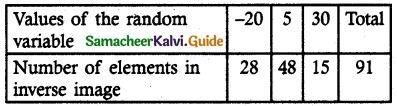Question 5.
A six-sided die is marked ‘2’ on one face, ‘3’ on two of its faces, and ‘4’ on the remaining three faces. The die is thrown twice. If X denotes the total score in two throws, find the values of the random variable and number of points in its inverse images.
Solution:
Let X be the random variable denotes the total score is two throws of a die.
Sample Space S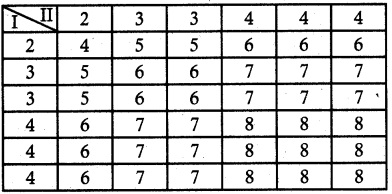n (S) = 36
X = {4, 5, 6, 7, 8}
From the sample space